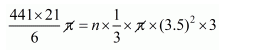# A metallic sphere of radius 10.5 cm

Question:

A metallic sphere of radius 10.5 cm is melted and thus recast into small cones, each of radius 3.5 cm and height 3 cm. Find how many cones are obtained.

Solution:

The radius of sphere = 10.5 cm

The volume of sphere

$=\frac{4}{3} \pi r^{3}$

$=\frac{4}{3} \pi \times(10.5)^{3}$

$=\frac{4}{3} \pi\left(\frac{21}{2}\right)^{3}$

$=\frac{4}{3} \pi \frac{441 \times 21}{8}$

$=\frac{441 \times 21}{6} \pi(\mathrm{cm})^{3}$

Let n be the number of cones obtained when the sphere is recast in to small cones, each of radius 3.5 and height 3 cm.

Then, volume of sphere = n × volume of cone$\frac{441 \times 21}{6}=n \times \frac{1}{3} \times \frac{7}{2} \times \frac{7}{2} \times 3$

$441 \times 21=\frac{n \times 49 \times 3}{2}$

$n=\frac{441 \times 21 \times 2}{49 \times 3}=126$

$n=126$

Hence, the no. of cones = 126